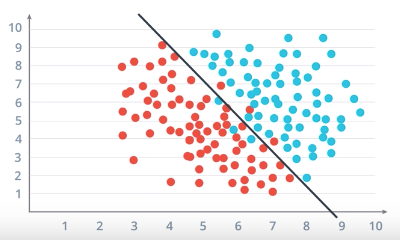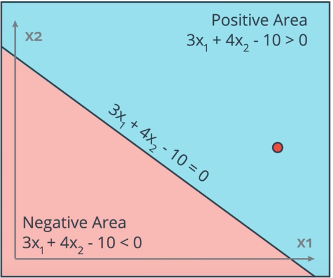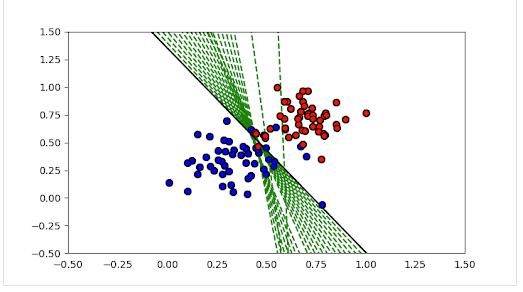# Perceptron Algorithm

These are my notes for Udacity’s Deep Learning with PyTorch course. Feel free to use them for your own reference if you like ;)

Note: I would like to give Udacity the credit for the material that I will be writing in this blog. These are my understanding from the course, also the images are taken from the course content.

A perceptron is like a single processing unit which can be used to form a linear classification/decision boundary between different classes.Linear Differentiating Boundary b/w Red & Blue data points

A perceptron is responsible to find a line of the form:

`WX + b = 0`

where

• X is dimension size vector which forms the variables (eg. X = [x1, x2]),
• W is the coefficient vector corresponding to each X (eg. W = [w1, w2]),
• b is a scalar bias value added to the line equation.

So, the equation for a 2-dimensional data point would be:

`WX + b = 0where, W = {w1, w2}X = {x1, x2}=> (w1 * x1) + (w2 * x2) + b = 0`

In a binary classifier, a data point is classified as either positive(blue)/negative(red) data point.

• Positive(Blue) data point if `WX + b ≥ 0`
• Negative(Red) data point if `WX + b < 0`

To find the value of WX + b, we simply replace the X values in the equation with the data point coordinates.

We use something called as Activation Function (here we can use Step Function) to give the Blue points a label 1 and Red points a label 0.

`def step_function(X):   if (WX + b) >= 0:     return 1 # Positive label   else:     return 0 # Negative label`

The question here is to know how to find the line of the equation i.e. the weights (W) and the Bias (b) such that the blue and red points are separated in such a way that maximum Red points are below the line and maximum Blue are above.

#### Here comes the use of Perceptron Trick!Red point is in the Positive (Blue) Region

In the above image the Negative point (Red) is in the Positive Region for our given line `(3 * x1) + (4 * x2) — 10 = 0`

Our objective is to somehow shift the line such that the red point comes below the new updated line, i.e., we need to bring the line closer to the Red point.

A neat trick for this is to do the following, suppose the red point has the coordinates (4,5):

1. For negative point in Positive region (i.e. WX + b ≥ 0):
• Take the line coefficients and subtract the misclassified point coordinate values from it.
• Also, subtract 1 from the bias value.

2. For positive point in Negative region (i.e. WX + b < 0):

• Take the line coefficients and add the misclassified point coordinate values into it.
• Also, add 1 to the bias value.

This way we shift the line towards the misclassified data point.

For example, here we’ll do:

`Old_W:      3    4    -10Coords:    -4   -5     -1   <- subtracting 1 from Bias as well         ------------------New_W    = -1   -1    -11`

But, there is a slight issue with this approach. This sudden change in coefficient values could lead to misclassifying many other points in the data set.

To avoid this issue, we update the weights slowly by introducing learning rate.

Learning rate ensures that the weights are updated slowly and hence there is a gradual shift of the line towards the desired region.

We multiple the learning rate with each of the coordinate values as well as the bias value.

In our previous example if learning rate = 0.1:

`Old_W:                      3.0    4.0    -10.0Coords * learning rate:    -0.4   -0.5     -0.1                        -------------------------New_W    =                  2.6    3.5     -9.9`

So, now our new line equation will be: `(2.6 * x1) + (3.5 * x2) - 9.9 = 0`

We do this process repeatedly to improve the weights for all the misclassified data points. The number of times we do this is known as the number of epochs.

So our Perceptron Algorithm would be as follows (α = learning rate):

`1. Start with random weights (W) and bias (b)2. For every misclassified point (p1, p2, …, pn):   2.1 if Red point (i.e. prediction = 1 `WX+b ≥ 0` but should be < 0)       For i <- 1 to n          update wi <- wi - α * xi          update b <- b - α`
`   2.2 if Blue point (i.e. prediction = 0 `WX+b <0` but should be >=0)       For i <- 1 to n          update wi <- wi + α * xi          update b <- b + α3. end`

The code function for this algorithm in python could be as follows:

You can visualise how the weights of the line got updated if you plot them at each epoch, you’ll find the output as below for some arbitrary dataset.Weight updations fit the line

I’ll be posting future blogs on PyTorch, Neural Networks, etc. from the course so stay tuned for more.

You can also get in touch with me on Twitter at @ShrimalAnubhav, look at my portfolio, or find me on LinkedIn and Facebook. I’d love to hear from you, any suggestion or feedbacks.